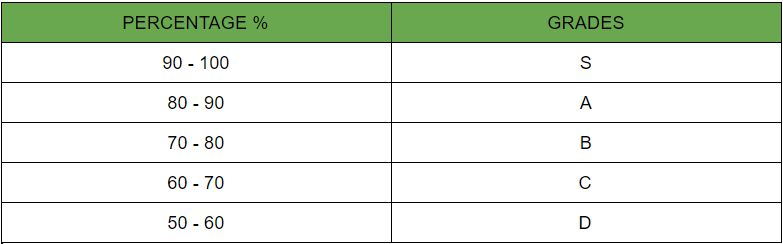Related Articles

# Java Program to Read a Grade & Display the Equivalent Description

• Last Updated : 31 Jul, 2021

Problem Statement: Numbers on a scale of 1 to 100 are randomly given the grade as the input from the user and then match the input grade of the user against the given cases to generate the desired output.

Real life Example

Consider a number on a scale of 1 to 100 are written on a piece of paper slips. Now person starts picking slips one by one and starts organizing them in such a manner that he starts putting slips in 10 different boxes prior deciding making 10 different pots in such a manner slips numbering 1-10 in ‘pot A’, 11-20 in ‘pot B’ and so on till labeling and inserting slips till all the slips are organized in labeled pots. He did this in order to easily figure out numbers written on slips.

So in technical terms In these ways machine get

• Take input from the user
• Match the grade using equal to the operator (==)
• Generate the equivalent description as the output

There are several methods starting from brute force(naive) to most optimal. Few are discussed below :

1.  Using the If-Else Method
2. Switch case Method

The grades are assigned using the followingApproach 1: If-Else Statement

Below is the implementation of the above approach

## Java

 `// Java Program to Read Grade & Displaying Equivalent Desc` `// Importing Classes/Files``import` `java.util.*;` `public` `class` `GFG {` `    ``// Main Driver Method``    ``public` `static` `void` `main(String[] args)``    ``{` `        ``// User is supposed to enter grade among them``        ``System.out.println(``            ``"Enter Grade varying from S,A,B,C,D"``);``        ``String grade = ``"A"``;` `        ``// Checking whether grade == "S" or not``        ``if` `(grade == ``"S"``) {``            ``System.out.println(``                ``"Student has scored between 90 to 100"``);``        ``}``        ``// Checking whether grade == "A" or not``        ``else` `if` `(grade == ``"A"``) {``            ``System.out.println(``                ``"Student has scored between 80 to 90"``);``        ``}``        ``// Checking whether grade == "B" or not``        ``else` `if` `(grade == ``"B"``) {``            ``System.out.println(``                ``"Student has scored between 70 to 80"``);``        ``}``        ``// Checking whether grade == "C" or not``        ``else` `if` `(grade == ``"C"``) {``            ``System.out.println(``                ``"Student has scored between 60 to 70"``);``        ``}``        ``// Checking whether grade == "D" or not``        ``else` `if` `(grade == ``"D"``) {``            ``System.out.println(``                ``"Student has scored between 50 to 60"``);``        ``}``        ``else` `{` `            ``// Printing message-user pressed some other key``            ``System.out.println(``                ``"The grade you entered is not valid!"``);``        ``}``    ``}``}`
Output
```Enter Grade varying from S,A,B,C,D
Student has scored between 80 to 90```

Approach 2: Switch Case

Below is the implementation of the above approach

## Java

 `// Java Program to Read a Grade & Display the Equivalent` `// Importing Classes/Files``import` `java.util.*;` `public` `class` `GFG {` `    ``//  Main driver method``    ``public` `static` `void` `main(String args[])``    ``{` `        ``// Random grade taken for consideration``        ``String grade = ``"W"``;` `        ``// Using Switch-Case.``        ``switch` `(grade) {``            ``// Checking whether grade == "S" or not.` `        ``case` `"S"``:``            ``System.out.println(``                ``"Student has scored between 90 to 100"``);``            ``break``;` `            ``// Checking whether grade == "A" or not.``        ``case` `"A"``:``            ``System.out.println(``                ``"Student has scored between 80 to 90"``);``            ``break``;` `            ``// Checking whether grade == "B" or not.``        ``case` `"B"``:``            ``System.out.println(``                ``"Student has scored between 70 to 80"``);``            ``break``;` `            ``// Checking whether grade == "C" or not.``        ``case` `"C"``:``            ``System.out.println(``                ``"Student has scored between 60 to 70"``);``            ``break``;` `            ``// Checking whether grade == "D" or not.``        ``case` `"D"``:``            ``System.out.println(``                ``"Student has scored between 50 to 60"``);``            ``break``;` `        ``default``:``            ``System.out.println(``                ``"The grade you entered is not valid!"``);``        ``}``    ``}``}`
Output
`The grade you entered is not valid!`

Attention reader! Don’t stop learning now. Get hold of all the important Java Foundation and Collections concepts with the Fundamentals of Java and Java Collections Course at a student-friendly price and become industry ready. To complete your preparation from learning a language to DS Algo and many more,  please refer Complete Interview Preparation Course.

My Personal Notes arrow_drop_up